Technical Article

# Isolated Logarithmic Amplifier Using HighLinearity Optocouplers

April 10, 2019 by Nelson Quek

## Logarithmic Amplifiers are used for isolation in circuit that includes analog and mixed signal ADC, present on circuit board with chipset, diode, etc.

Logarithmic amplifiers with its log functionality are useful for compressing wide dynamic range signals whereby the measured quantities are in decibels (dB) and they are used in a wide variety of applications such as video, medical, and test and measurement systems. Logarithmic amplifiers can be built to a compact, easy to use and cost-effective circuits suitable for certain analog designs.

Because of the high voltage presence in industrial applications, it is necessary to protect equipment and personnel operating the motors through galvanic isolation. HCNR201/200 can be used for current sensing and voltage monitoring in motor control drives, switching power supplies and inverter systems.

The HCNR201/200 analog optocouplers are commonly added to isolate the analog signal in the front end module of an application circuitry. The optocoupler will be placed between the analog input and the A/D converter to provide isolation of the analog input from the mixed signal ADC and other digital circuitries.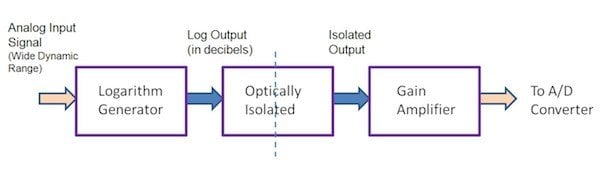## Optical Isolation Using HCNR201/200

Isolation using Broadcom optocouplers will protect the control part (such as the MCUs) from the high voltage side of the IGBTs, in the case of malfunctioning of the IGBTs for motor drives.

Broadcom high linearity optocoupler, HCNR201/200 provides an excellent solution in isolating analog signals in various applications that require good stability, linearity, bandwidth, and low cost. HCNR201/200 consists of 1 LED and two closely matched photodiodes (PD1 and PD2). The important parameter for HCNR201/200 is the transfer gain and it determines how closely matched are the two photodiodes. HCNR201 is with tighter transfer gain of 5% and HCNR200 is with transfer gain of 15%. With the tight transfer gain governed by the photodiodes, Broadcom high linearity optocouplers virtually eliminate the nonlinearities and drift characteristics of the LED, with HCNR201 achieving 0.07% non-linearity over temperature. An example of an isolated gain amplifier circuitry using the HCNR201/200 is illustrated below and the HCNR201/200 is connected in photovoltaic mode as the voltage across the photodiodes are essentially zero volts.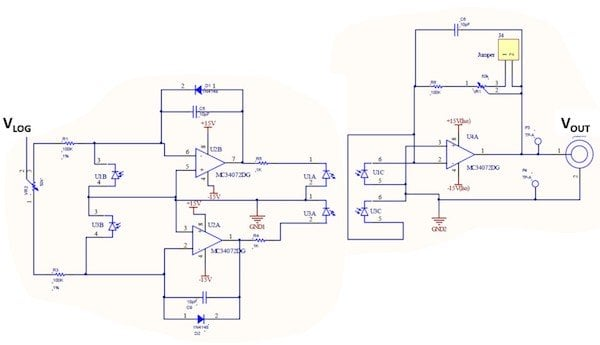Figure 1: HCNR201/200 Bipolar Analog Input Isolation circuitry (U1A, U1B, U1C and U3A, U3B, U3C as 2 HCNR optocouplers respectively)

The above bipolar input voltage circuit uses either two HCNR201 or HCNR200 optocouplers (U1 and U3). The top half of the circuit consisting of Photodiode (U1B), R1, D1, C5, R5, and LED (U1A) is for the positive input voltages. The lower half of the circuit consisting of Photodiode (U3B), R3, D2, C9, and R4 and LED (U3A) is for the negative input voltages. The diodes D1 and D2 help reduce crossover distortion by keeping both amplifiers active during both positive and negative portions of the input signal. Balance control VR2 at the input can be used to adjust the relative gain for the positive and negative input voltages. The gain control VR1 can be used to adjust the overall transfer gain of the amplifier. The capacitors C5, C6, and C9 are the compensation capacitors for stability.

................ (1)

................ (2)

K3 is the transfer gain of HCNR201/200.

IPD2 is the current flowing through photodiode, PD2 and IPD1 is the current flowing through photodiode, PD1.

Therefore, if R1 + VR2 = R6 + VR1, then the gain of the amplifier will be unity and output signal will follow the input signal.

## Logarithmic Generator

For applications involving signals with large dynamic range, it’s difficult to deal with both small and large amplitude signals. Thus, it will require a log amplifier for signal compression. A simple circuit for the logarithmic function generator is shown in Figure 2. The circuit is constructed with a pair of matched transistors, Q1, Q2, and operational amplifiers. Transistors Q1 and Q2 will serve as the feedback element for the inverting operational amplifier. The circuit output, VLOG is the logarithmical value of the input signal, VIN. With the zener diode LM329, the collector current for Q2 is fixed and therefore, VBE2 is fixed. Only VBE1 will be affected by input signal, VIN.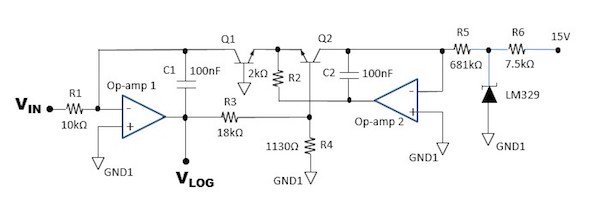Figure 2: Logarithmic Generator Circuit

The output voltage, VLOG is proportional to the difference in the base-emitter voltages of Q1 and Q2.

................ (1)

For different collector currents at Q1 and Q2, the VBE difference is governed by

................ (2)

Substituting equation (2) to (1), with a difference of VBE2 and VBE1 as ΔVBE

................ (3)

ICQ1 and ICQ2 equations are as below:

................ (4)

................ (5)

Substituting equation (4) and (5) into (3) :

................ (6)

With Vz of LM329 = 6.9V, and R5 = 681kΩ, R1 = 10kΩ, the logarithmic amplifier circuit gain is set by the R3 and R4 divider, (R3+R4) / R4 to a factor of 1V/decade. kT / q is equal to 0.02568V @ room temperature of 25°C where k is the Boltzmann’s constant (1.38064852 x 10-23 m2.kg.s-2.K-1), T is the temperature in Kelvin and q is the charge of an electron (1.60217662 x 10-19 coulombs).

VBE1 is the base-emitter voltage for the bipolar transistor, Q1 and VBE2 is the base-emitter voltage for the bipolar transistor, Q2. ICQ1 is the collector current for the bipolar transistor, Q1. ICQ2 is the collector current for the bipolar transistor, Q2. VZ is the zener diode voltage.

### Evaluation Board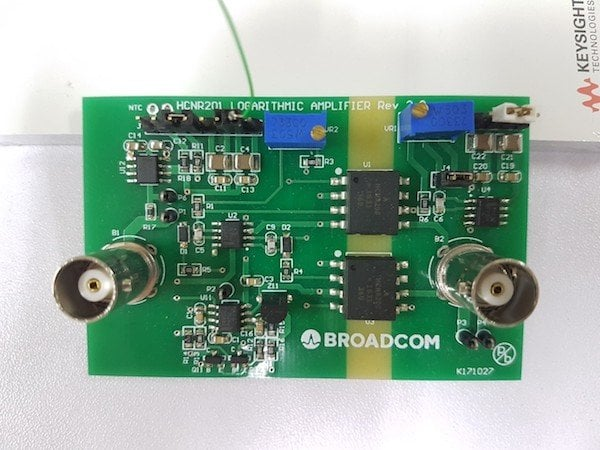Above evaluation board is built by cascading Figure 2 and Figure 1 together. Component U1 and U3 are the two HCNR201/200 optocouplers providing isolation between the input and output circuitry.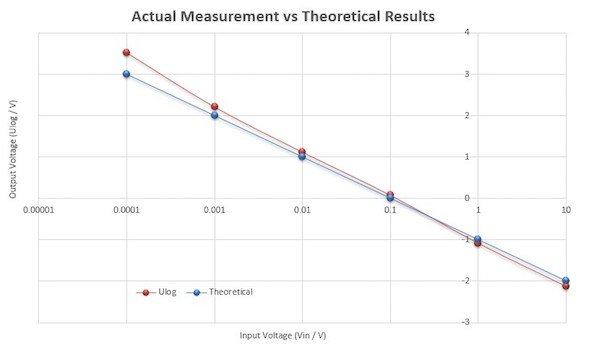Figure 3: Vout vs Vin plot (DC input signal)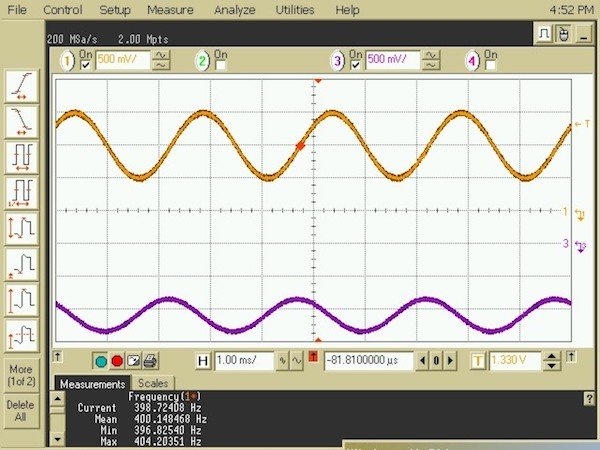Figure 4: Vout vs Vin plot (AC input signal)

## Results: DC Performance

Figure 3 shows the plot of output voltage vs input voltage for different DC input voltages ranging from 0.1mV to 10V. Theoretical results are computed based on Equation 6. Actual Measurement result is based on the evaluation board built from the cascaded isolated and logarithmic circuits in Figure 1 and 2 respectively.

## Results: AC Performance

Figure 4 shows the output voltage signal (purple waveform) with 1Vp-p AC input signal (orange waveform). Input AC signal is riding on 1Vdc, therefore from Figure 3 plot, output is with -1Vdc. Based on -3dB cutoff frequency, and output signal is found to be at 0.5Vp-p which is at half of the input signal. The bandwidth (-3dB) of this circuit is therefore, 400Hz.

For AC performance, the instantaneous input AC signal level must not be less than or equal to 0V as the logarithmic value of 0V and the negative input value is undefined. If input AC signal of less than or equal to 0V is applied, the logarithmic value will be clipped at the maximum value.

## Summary

Logarithmic amplifiers applications include digital communication systems, analytical, medical test and instrumentation whereby safety isolation plays an important role as well. These types of industrial applications measure the physical quantities over a wide dynamic range and therefore use log amps to match the dynamic output to the linear input range of the signal. HCNR201/200 high linearity optocouplers will provide optical isolation to ensure data integrity and to protect operators from high voltages.

### References

 A galvanic isolated evaluation of a NTC thermistor using a loga-rithmic amplifier, Yevgen Polonskiy, - Antalya, Turkey 21-24 Sept 2014.

 AN-30 Log Converters, Application Report, Texas Instruments - May, 2013

 HCNR201, HCNR200 High Linearity Analog Optocouplers, Data-sheets AV02-0886EN, Avago Technologies. Jul 1, 2014

 HCNR201/200, High-Linearity Analog Optocouplers Evaluation Board User’s Manual, AV02-1134EN, Avago Technologies. Apr 2, 2008